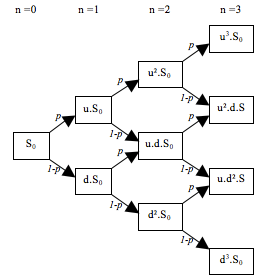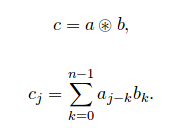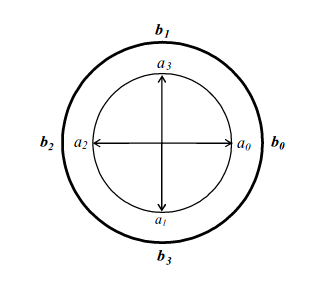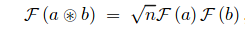# Buy High, Sell Low: Fast Fourier Transforms in Options

Some of you engineering types should have learned about Fourier transforms. Some of you might even know that they can be done quickly in what are called fast Fourier Transforms (FFT). But I bet you didn’t know how FFTs are used everyday in finance to find the value of an option. We will keep the math to a minimum for the purposes of this article.

We start with an option, a financial instrument used to “bet” on the changing price of an asset such as stock in a company. The true mechanic of this agreement is the option holder’s ability to buy (call) or sell (put) the asset at a constant price over the life of the contract, regardless of the real time movement in the asset’s value. For example, if you expect the price of Apple’s stock to increase, a call option would give you ability to purchase a share during the life of the contract for the price specified when it was issued. The future price, or strike price, will be higher than the option price, giving you the ability to make a profit equal to the difference between those values. Put options work similarly but for the case in which you expect the value of an asset to decrease.

The use of options begs for a better way to predict the possible values of an options contract at the strike time. Using a bit of probability and statistics we can generate a binomial tree of possible price paths for any asset.

We assume that the price of the asset can go either up or down by fixed factors u>1 and d<1 such that u and d are multiplicative inverses of each other. If we obtain an options contract at n=0 for the price of S0 that is exercised at n=3 time steps when the strike price is K, we can find the difference K – S3 and work backwards from the last time step to determine the value of the option at each parent node based on what are known as state prices for the up and down movement.At this point, I can only hope my audience has been reduced to at least the EE’s and other mathe-masochists, yearning to know how Fourier transforms, let alone fast Fourier transforms, fit into this picture. We begin with a simplified version of what is known as cyclic or circular convolution where a, b and c are n dimensional vectors:This process is best thought of as two concentric circles: one with the entries of b in order going counterclockwise and the other with the entries of a, in order, clockwise. By summing the scalar multiplications of each aligned entries, we have the jth entry of c where aj and b0 are aligned. By rotating the a circle counterclockwise to the next alignment and repeating the sum of products for all n entries, we obtain the complete c vector. If we take the a vector to be the striked value of the option at the last time step and the b vector as the up and down state prices padded with zeroes, performing the cyclic convolution for each time step gives us the same result as the working backwards process in Binomial Options. The real magic happens when we see how the Fourier Transformation turns this complex, time consuming algorithm into a simple product.Assuming you won’t be caught doing these calculations by hand, they are best done using MATLAB, Mathematica or other software containing prepackaged algorithms to perform the transformation in its fast form. Unfortunately I won’t have enough room to go into great detail on FFT, but I think I’ve made my point. ◊

I’m sure some of you will need more than a one line proof so to speak. Read more on all of this below:

-Cerný, A. (2004). Introduction to FFT in Finance. Princeton University Press.

-Cox, J. C.; Ross, S. A.; Rubinstein, M. (1979). Option Pricing: A Simplified Approach. Journal of Financial Economics.